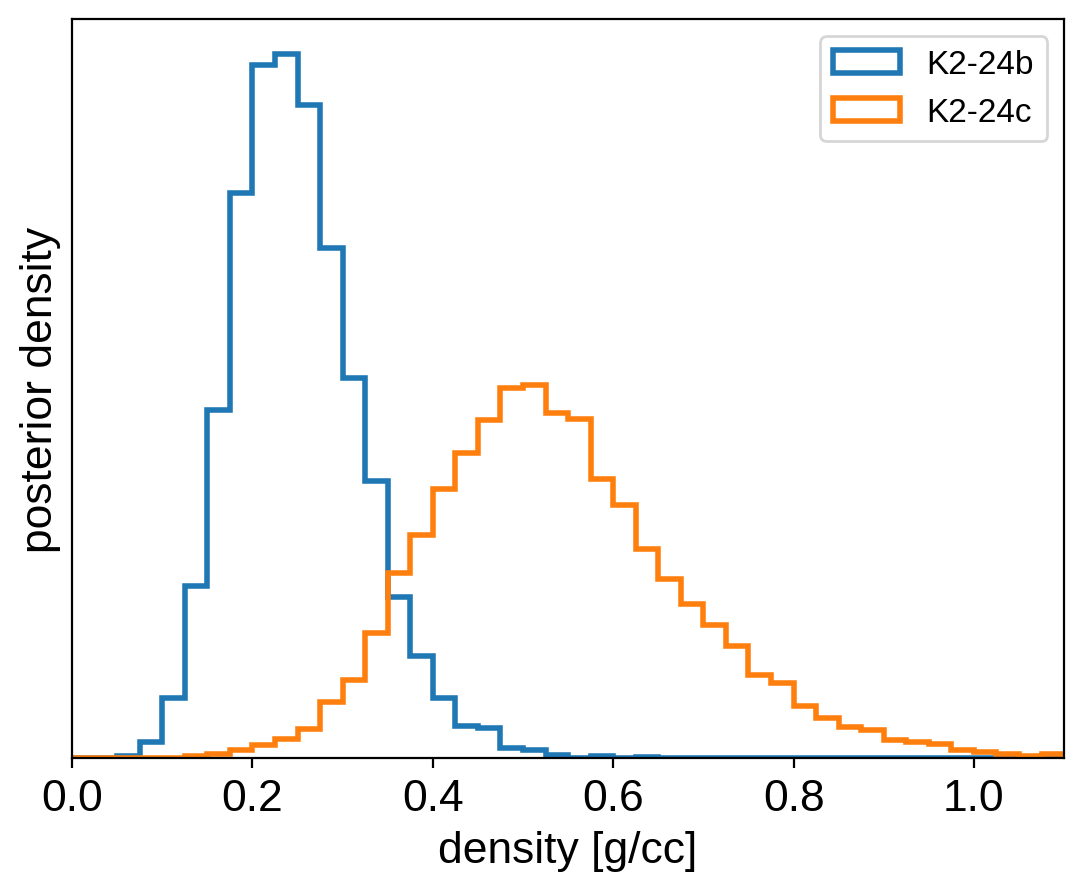Note

This tutorial was generated from an IPython notebook that can be downloaded here.

# Putting it all together¶

In this tutorial, we will combine many of the previous tutorials to perform a fit of the K2-24 system using the K2 transit data and the RVs from Petigura et al. (2016). This is the same system that we fit in the Radial velocity fitting tutorial and we’ll combine that model with the transit model from the Transit fitting tutorial and the Gaussian Process noise model from the Gaussian process models for stellar variability tutorial.

## Datasets and initializations¶

To get started, let’s download the relevant datasets. First, the transit light curve from Everest:

import numpy as np
import matplotlib.pyplot as plt

from astropy.io import fits
from scipy.signal import savgol_filter

lc_url = "https://archive.stsci.edu/hlsps/everest/v2/c02/203700000/71098/hlsp_everest_k2_llc_203771098-c02_kepler_v2.0_lc.fits"
with fits.open(lc_url) as hdus:
lc = hdus.data

# Work out the exposure time
texp = lc_hdr["FRAMETIM"] * lc_hdr["NUM_FRM"]
texp /= 60.0 * 60.0 * 24.0

m = (np.arange(len(lc)) > 100) & np.isfinite(lc["FLUX"]) & np.isfinite(lc["TIME"])
bad_bits = [1, 2, 3, 4, 5, 6, 7, 8, 9, 11, 12, 13, 14, 16, 17]
qual = lc["QUALITY"]
m &= qual & 2 ** (b - 1) == 0

# Convert to parts per thousand
x = lc["TIME"][m]
y = lc["FLUX"][m]
mu = np.median(y)
y = (y / mu - 1) * 1e3

# Identify outliers
m = np.ones(len(y), dtype=bool)
for i in range(10):
y_prime = np.interp(x, x[m], y[m])
smooth = savgol_filter(y_prime, 101, polyorder=3)
resid = y - smooth
sigma = np.sqrt(np.mean(resid ** 2))
m0 = np.abs(resid) < 3 * sigma
if m.sum() == m0.sum():
m = m0
break
m = m0

m = resid < 3 * sigma

# Shift the data so that the K2 data start at t=0. This tends to make the fit
# better behaved since t0 covaries with period.
x_ref = np.min(x[m])
x -= x_ref

# Plot the data
plt.plot(x, y, "k", label="data")
plt.plot(x, smooth)
plt.plot(x[~m], y[~m], "xr", label="outliers")
plt.legend(fontsize=12)
plt.xlim(x.min(), x.max())
plt.xlabel("time")
plt.ylabel("flux")

# Make sure that the data type is consistent
x = np.ascontiguousarray(x[m], dtype=np.float64)
y = np.ascontiguousarray(y[m], dtype=np.float64)
smooth = np.ascontiguousarray(smooth[m], dtype=np.float64)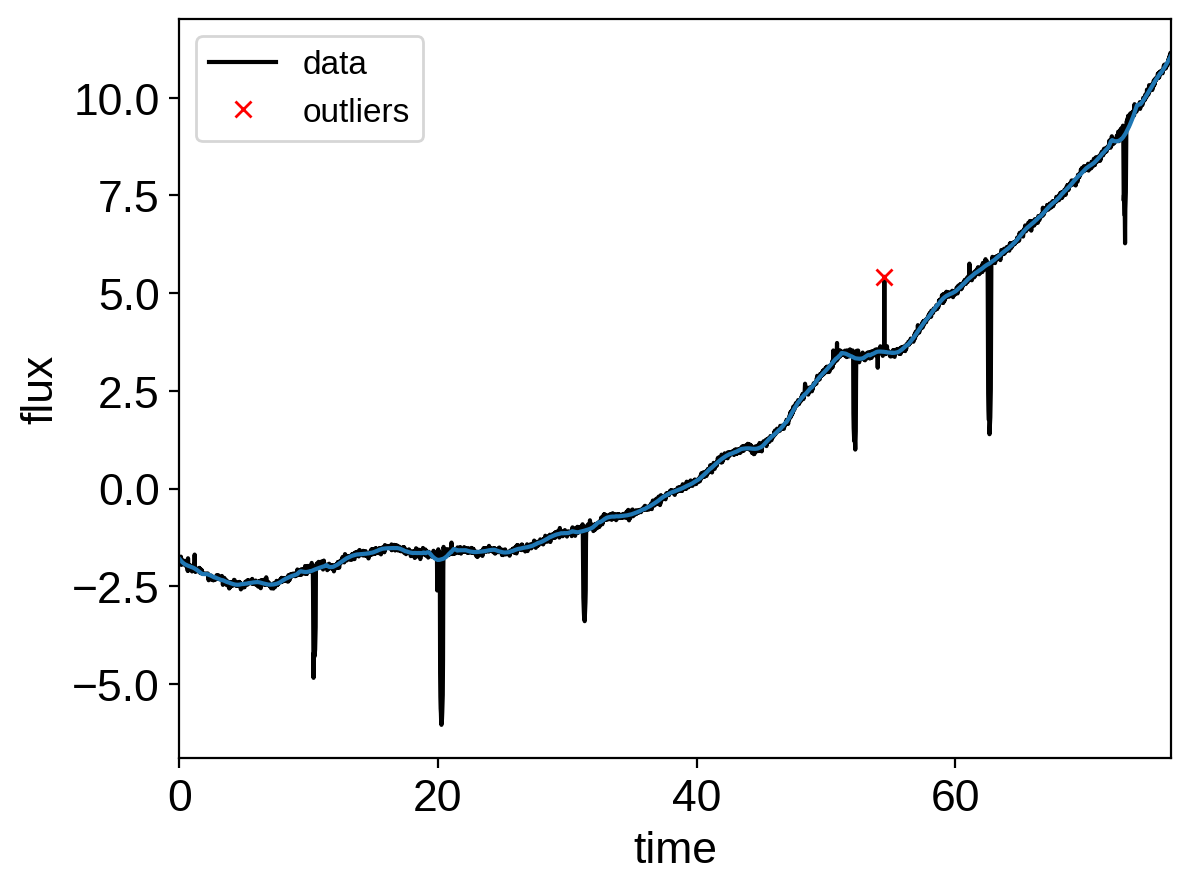import pandas as pd

# Don't forget to remove the time offset from above!
x_rv = np.array(data.t) - x_ref
y_rv = np.array(data.vel)
yerr_rv = np.array(data.errvel)

plt.errorbar(x_rv, y_rv, yerr=yerr_rv, fmt=".k")
plt.xlabel("time [days]")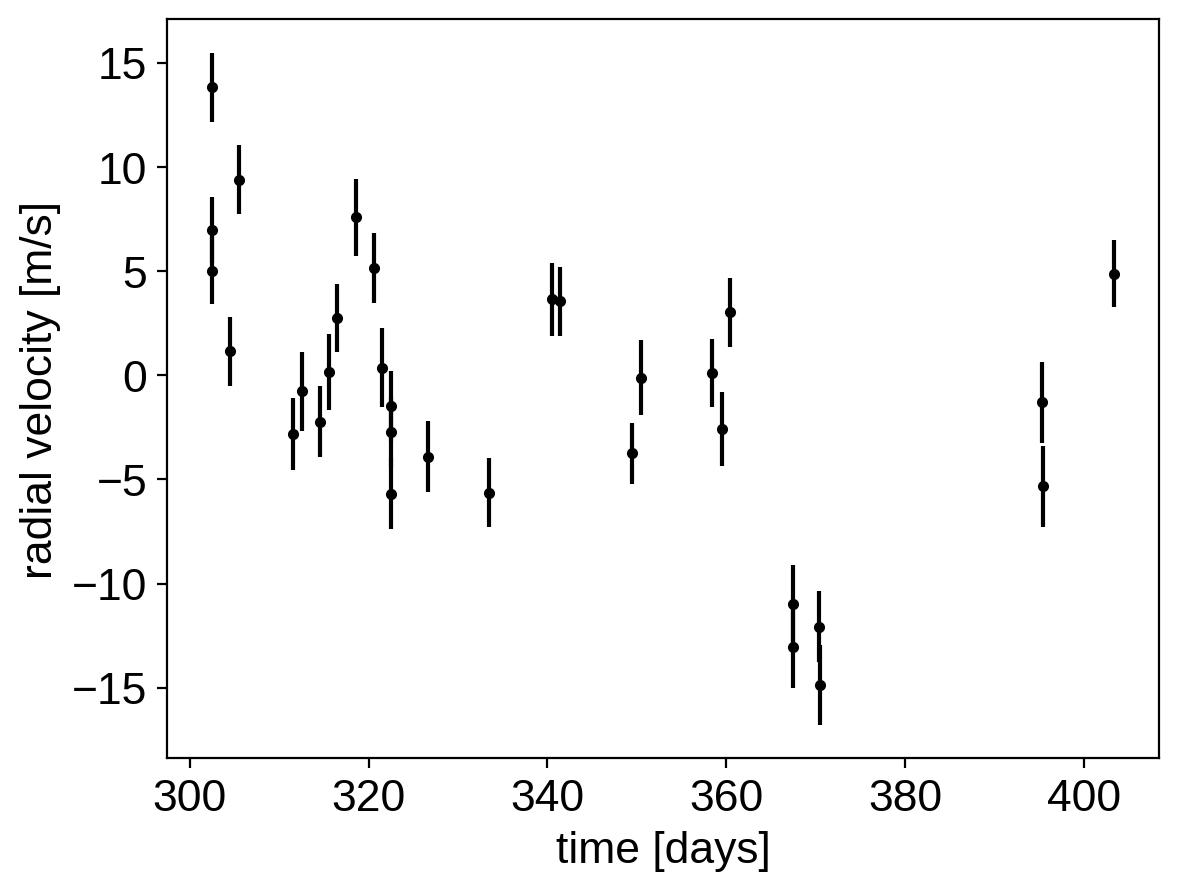We can initialize the transit parameters using the box least squares periodogram from AstroPy. (Note: you’ll need AstroPy v3.1 or more recent to use this feature.) A full discussion of transit detection and vetting is beyond the scope of this tutorial so let’s assume that we know that there are two periodic transiting planets in this dataset.

from astropy.timeseries import BoxLeastSquares

m = np.zeros(len(x), dtype=bool)
period_grid = np.exp(np.linspace(np.log(5), np.log(50), 50000))
bls_results = []
periods = []
t0s = []
depths = []

# Compute the periodogram for each planet by iteratively masking out
# transits from the higher signal to noise planets. Here we're assuming
# that we know that there are exactly two planets.
for i in range(2):
bls = BoxLeastSquares(x[~m], y[~m] - smooth[~m])
bls_power = bls.power(period_grid, 0.1, oversample=20)
bls_results.append(bls_power)

# Save the highest peak as the planet candidate
index = np.argmax(bls_power.power)
periods.append(bls_power.period[index])
t0s.append(bls_power.transit_time[index])
depths.append(bls_power.depth[index])

# Mask the data points that are in transit for this candidate
m |= bls.transit_mask(x, periods[-1], 0.5, t0s[-1])


Let’s plot the initial transit estimates based on these periodograms:

fig, axes = plt.subplots(len(bls_results), 2, figsize=(15, 10))

for i in range(len(bls_results)):
# Plot the periodogram
ax = axes[i, 0]
ax.axvline(np.log10(periods[i]), color="C1", lw=5, alpha=0.8)
ax.plot(np.log10(bls_results[i].period), bls_results[i].power, "k")
ax.annotate(
"period = {0:.4f} d".format(periods[i]),
(0, 1),
xycoords="axes fraction",
xytext=(5, -5),
textcoords="offset points",
va="top",
ha="left",
fontsize=12,
)
ax.set_ylabel("bls power")
ax.set_yticks([])
ax.set_xlim(np.log10(period_grid.min()), np.log10(period_grid.max()))
if i < len(bls_results) - 1:
ax.set_xticklabels([])
else:
ax.set_xlabel("log10(period)")

# Plot the folded transit
ax = axes[i, 1]
p = periods[i]
x_fold = (x - t0s[i] + 0.5 * p) % p - 0.5 * p
m = np.abs(x_fold) < 0.4
ax.plot(x_fold[m], y[m] - smooth[m], ".k")

# Overplot the phase binned light curve
bins = np.linspace(-0.41, 0.41, 32)
denom, _ = np.histogram(x_fold, bins)
num, _ = np.histogram(x_fold, bins, weights=y - smooth)
denom[num == 0] = 1.0
ax.plot(0.5 * (bins[1:] + bins[:-1]), num / denom, color="C1")

ax.set_xlim(-0.4, 0.4)
ax.set_ylabel("relative flux [ppt]")
if i < len(bls_results) - 1:
ax.set_xticklabels([])
else:
ax.set_xlabel("time since transit")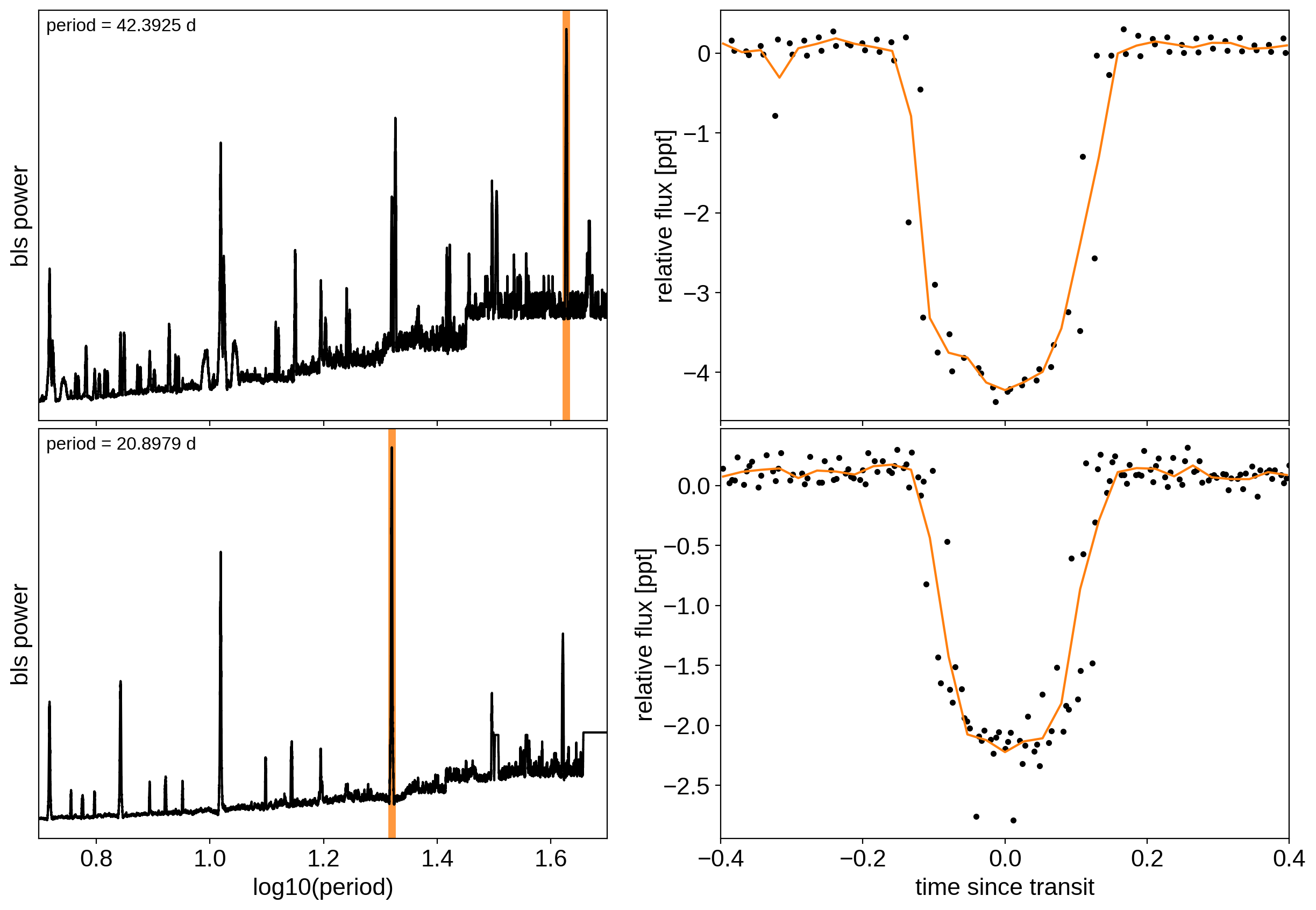The discovery paper for K2-24 (Petigura et al. (2016)) includes the following estimates of the stellar mass and radius in Solar units:

M_star_petigura = 1.12, 0.05
R_star_petigura = 1.21, 0.11


Finally, using this stellar mass, we can also estimate the minimum masses of the planets given these transit parameters.

import exoplanet as xo
import astropy.units as u

msini = xo.estimate_minimum_mass(
periods, x_rv, y_rv, yerr_rv, t0s=t0s, m_star=M_star_petigura
)
msini = msini.to(u.M_earth)
print(msini)

[32.80060146 23.89885976] earthMass


## A joint transit and radial velocity model in PyMC3¶

Now, let’s define our full model in PyMC3. There’s a lot going on here, but I’ve tried to comment it and most of it should be familiar from the previous tutorials (Radial velocity fitting, Transit fitting, Scalable Gaussian processes in PyMC3, and Gaussian process models for stellar variability). In this case, I’ve put the model inside a model “factory” function because we’ll do some sigma clipping below.

import pymc3 as pm
import theano.tensor as tt

t_rv = np.linspace(x_rv.min() - 5, x_rv.max() + 5, 1000)

with pm.Model() as model:

# Parameters for the stellar properties
mean = pm.Normal("mean", mu=0.0, sd=10.0)
BoundedNormal = pm.Bound(pm.Normal, lower=0, upper=3)
m_star = BoundedNormal("m_star", mu=M_star_petigura, sd=M_star_petigura)
r_star = BoundedNormal("r_star", mu=R_star_petigura, sd=R_star_petigura)

# Orbital parameters for the planets
logm = pm.Normal("logm", mu=np.log(msini.value), sd=1, shape=2)
logP = pm.Normal("logP", mu=np.log(periods), sd=1, shape=2)
t0 = pm.Normal("t0", mu=np.array(t0s), sd=1, shape=2)
logr = pm.Normal(
"logr",
mu=0.5 * np.log(1e-3 * np.array(depths)) + np.log(R_star_petigura),
sd=1.0,
shape=2,
)
r_pl = pm.Deterministic("r_pl", tt.exp(logr))
ror = pm.Deterministic("ror", r_pl / r_star)
b = xo.distributions.ImpactParameter("b", ror=ror, shape=2)

ecc = xo.distributions.eccentricity.vaneylen19(
"ecc", multi=True, shape=2, testval=np.array([0.01, 0.01])
)
omega = xo.distributions.Angle("omega", shape=2)

# RV jitter & a quadratic RV trend
logs_rv = pm.Normal("logs_rv", mu=np.log(np.median(yerr_rv)), sd=5)
trend = pm.Normal("trend", mu=0, sd=10.0 ** -np.arange(3)[::-1], shape=3)

# Transit jitter & GP parameters
logw0 = pm.Normal("logw0", mu=0.0, sd=10)

# Tracking planet parameters
period = pm.Deterministic("period", tt.exp(logP))
m_pl = pm.Deterministic("m_pl", tt.exp(logm))

# Orbit model
orbit = xo.orbits.KeplerianOrbit(
r_star=r_star,
m_star=m_star,
period=period,
t0=t0,
b=b,
m_planet=xo.units.with_unit(m_pl, msini.unit),
ecc=ecc,
omega=omega,
)

# Compute the model light curve using starry
light_curves = (
xo.LimbDarkLightCurve(u_star).get_light_curve(
)
* 1e3
)
light_curve = pm.math.sum(light_curves, axis=-1) + mean
pm.Deterministic("light_curves", light_curves)

# GP model for the light curve
kernel = xo.gp.terms.SHOTerm(log_Sw4=logSw4, log_w0=logw0, Q=1 / np.sqrt(2))
pm.Deterministic("gp_pred", gp.predict())

# Set up the RV model and save it as a deterministic
# for plotting purposes later

# Define the background RV model
A = np.vander(x_rv - 0.5 * (x_rv.min() + x_rv.max()), 3)
bkg = pm.Deterministic("bkg", tt.dot(A, trend))

# The likelihood for the RVs
rv_model = pm.Deterministic("rv_model", tt.sum(vrad, axis=-1) + bkg)
err = tt.sqrt(yerr_rv ** 2 + tt.exp(2 * logs_rv))
pm.Normal("obs", mu=rv_model, sd=err, observed=y_rv)

A_pred = np.vander(t_rv - 0.5 * (x_rv.min() + x_rv.max()), 3)
bkg_pred = pm.Deterministic("bkg_pred", tt.dot(A_pred, trend))

# Fit for the maximum a posteriori parameters, I've found that I can get
# a better solution by trying different combinations of parameters in turn
if start is None:
start = model.test_point
map_soln = xo.optimize(start=start, vars=[trend])
map_soln = xo.optimize(start=map_soln, vars=[logs2])
map_soln = xo.optimize(start=map_soln, vars=[logr, b])
map_soln = xo.optimize(start=map_soln, vars=[logP, t0])
map_soln = xo.optimize(start=map_soln, vars=[logs2, logSw4])
map_soln = xo.optimize(start=map_soln, vars=[logw0])
map_soln = xo.optimize(start=map_soln)

return model, map_soln

model0, map_soln0 = build_model()

optimizing logp for variables: [trend]
12it [00:11,  1.09it/s, logp=-8.276752e+03]
message: Optimization terminated successfully.
logp: -8290.066560739391 -> -8276.751877588842
optimizing logp for variables: [logs2]
15it [00:01,  9.91it/s, logp=2.110025e+03]
message: Optimization terminated successfully.
logp: -8276.751877588842 -> 2110.02456128196
optimizing logp for variables: [b, logr, r_star]
105it [00:01, 56.52it/s, logp=2.689040e+03]
message: Desired error not necessarily achieved due to precision loss.
logp: 2110.02456128196 -> 2689.0397283218103
optimizing logp for variables: [t0, logP]
79it [00:01, 42.45it/s, logp=3.244872e+03]
message: Desired error not necessarily achieved due to precision loss.
logp: 2689.039728321822 -> 3244.8715047912565
optimizing logp for variables: [logSw4, logs2]
13it [00:01,  7.96it/s, logp=3.899740e+03]
message: Optimization terminated successfully.
logp: 3244.8715047912547 -> 3899.7404342927084
optimizing logp for variables: [logw0]
12it [00:01,  6.65it/s, logp=4.004137e+03]
message: Optimization terminated successfully.
logp: 3899.7404342927084 -> 4004.1373334508944
optimizing logp for variables: [logSw4, logw0, logs2, trend, logs_rv, omega, ecc_frac, ecc_sigma_rayleigh, ecc_sigma_gauss, ecc, b, logr, t0, logP, logm, r_star, m_star, u_star, mean]
339it [00:04, 81.21it/s, logp=4.775494e+03]
message: Desired error not necessarily achieved due to precision loss.
logp: 4004.137333450869 -> 4775.494114320922


Now let’s plot the map radial velocity model.

def plot_rv_curve(soln):
fig, axes = plt.subplots(2, 1, figsize=(10, 5), sharex=True)

ax = axes
ax.errorbar(x_rv, y_rv, yerr=yerr_rv, fmt=".k")
ax.plot(t_rv, soln["bkg_pred"], ":k", alpha=0.5)
ax.plot(t_rv, soln["rv_model_pred"], label="model")
ax.legend(fontsize=10)

ax = axes
err = np.sqrt(yerr_rv ** 2 + np.exp(2 * soln["logs_rv"]))
ax.errorbar(x_rv, y_rv - soln["rv_model"], yerr=err, fmt=".k")
ax.axhline(0, color="k", lw=1)
ax.set_ylabel("residuals [m/s]")
ax.set_xlim(t_rv.min(), t_rv.max())
ax.set_xlabel("time [days]")

plot_rv_curve(map_soln0)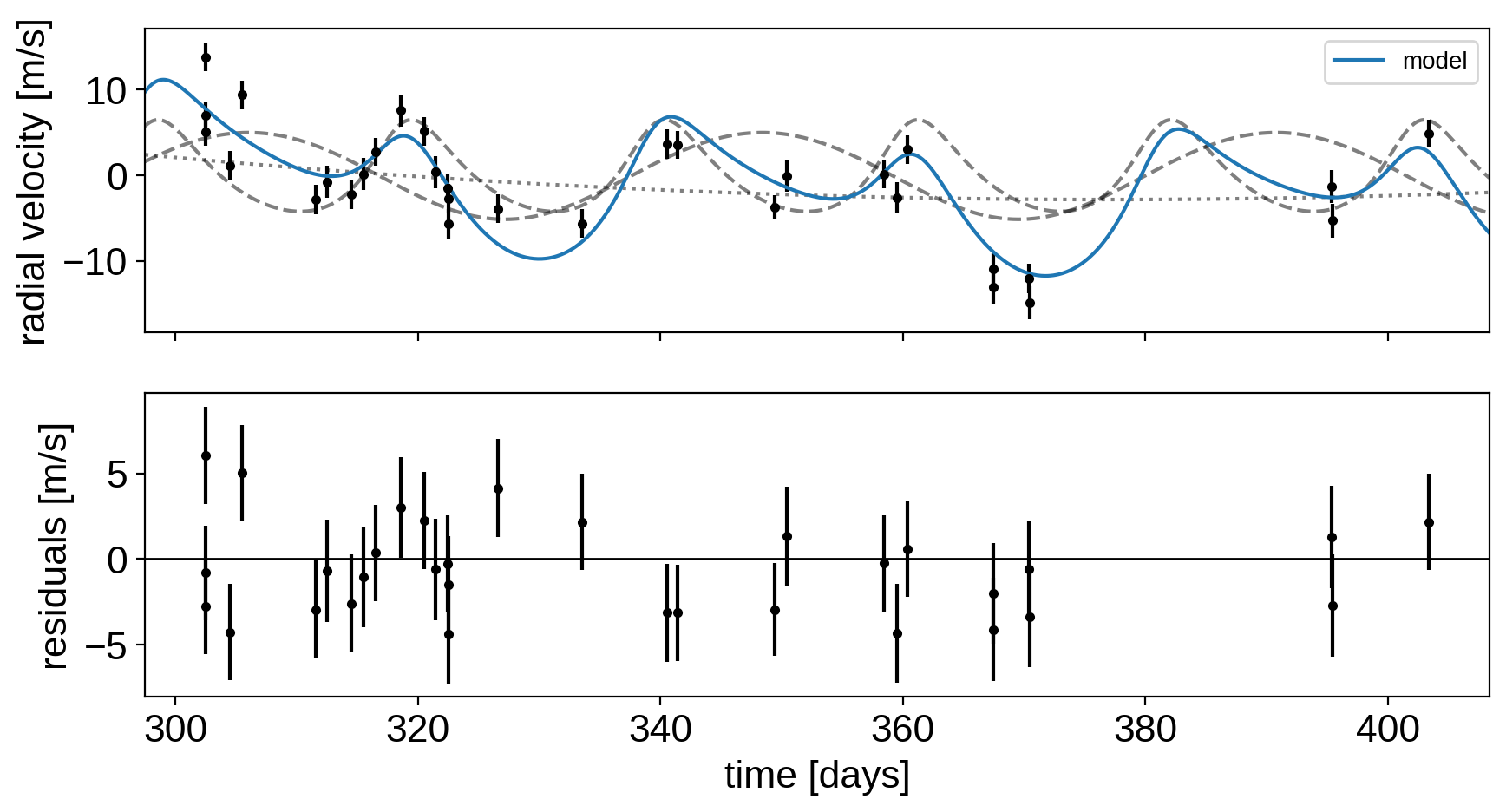That looks pretty similar to what we got in Radial velocity fitting. Now let’s also plot the transit model.

def plot_light_curve(soln, mask=None):

fig, axes = plt.subplots(3, 1, figsize=(10, 7), sharex=True)

ax = axes
gp_mod = soln["gp_pred"] + soln["mean"]
ax.legend(fontsize=10)
ax.set_ylabel("relative flux [ppt]")

ax = axes
for i, l in enumerate("bc"):
mod = soln["light_curves"][:, i]
ax.legend(fontsize=10, loc=3)
ax.set_ylabel("de-trended flux [ppt]")

ax = axes
mod = gp_mod + np.sum(soln["light_curves"], axis=-1)
ax.axhline(0, color="#aaaaaa", lw=1)
ax.set_ylabel("residuals [ppt]")
ax.set_xlabel("time [days]")

return fig

plot_light_curve(map_soln0);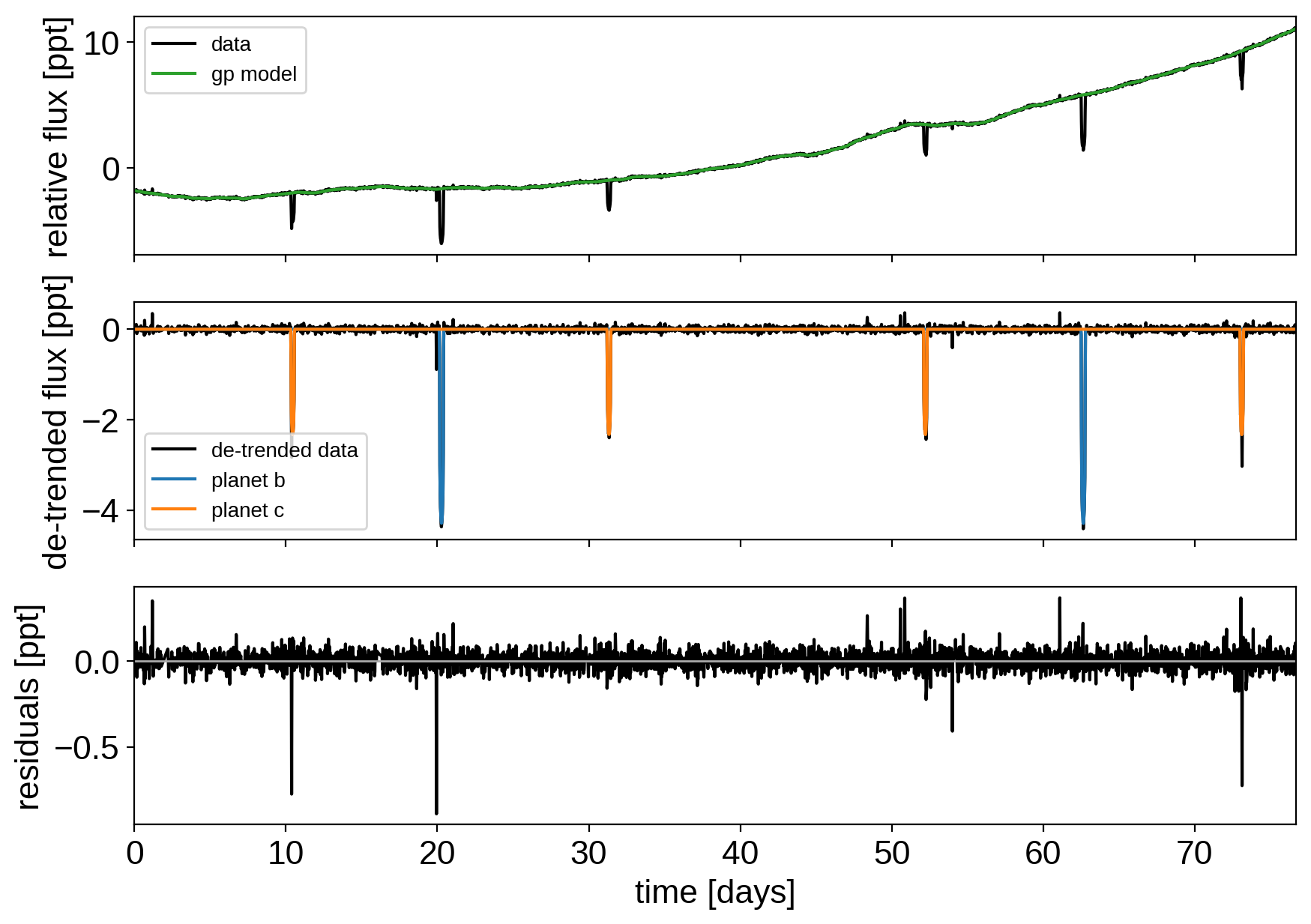There are still a few outliers in the light curve and it can be useful to remove those before doing the full fit because both the GP and transit parameters can be sensitive to this.

## Sigma clipping¶

To remove the outliers, we’ll look at the empirical RMS of the residuals away from the GP + transit model and remove anything that is more than a 7-sigma outlier.

mod = (
map_soln0["gp_pred"]
+ map_soln0["mean"]
+ np.sum(map_soln0["light_curves"], axis=-1)
)
resid = y - mod
rms = np.sqrt(np.median(resid ** 2))
mask = np.abs(resid) < 7 * rms

plt.plot(x, resid, "k", label="data")
plt.axhline(0, color="#aaaaaa", lw=1)
plt.ylabel("residuals [ppt]")
plt.xlabel("time [days]")
plt.legend(fontsize=12, loc=4)
plt.xlim(x.min(), x.max());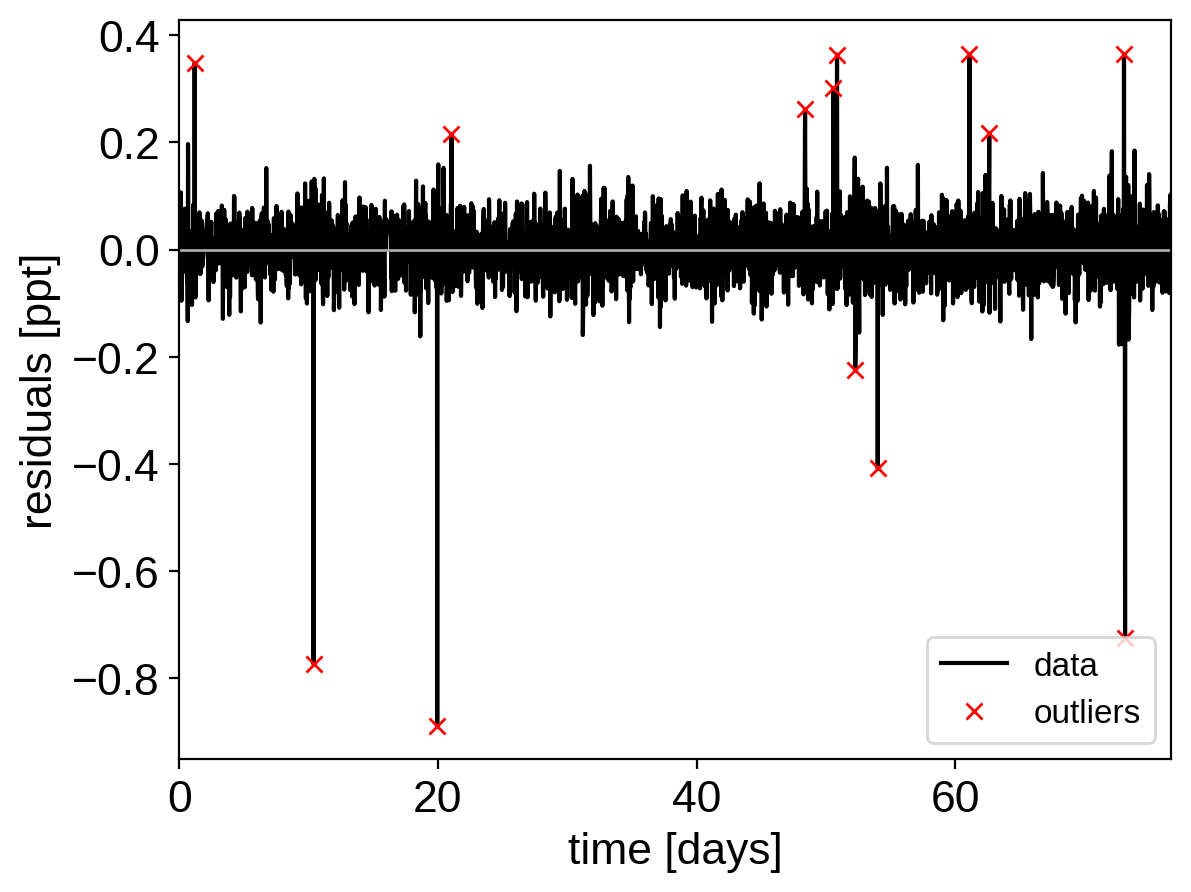That looks better. Let’s re-build our model with this sigma-clipped dataset.

model, map_soln = build_model(mask, map_soln0)

optimizing logp for variables: [trend]
5it [00:01,  2.95it/s, logp=5.225381e+03]
message: Optimization terminated successfully.
logp: 5225.381218190808 -> 5225.381218190808
optimizing logp for variables: [logs2]
9it [00:01,  6.10it/s, logp=5.307429e+03]
message: Optimization terminated successfully.
logp: 5225.381218190808 -> 5307.42938442338
optimizing logp for variables: [b, logr, r_star]
26it [00:01, 16.44it/s, logp=5.318396e+03]
message: Optimization terminated successfully.
logp: 5307.42938442338 -> 5318.395979595314
optimizing logp for variables: [t0, logP]
165it [00:02, 67.62it/s, logp=5.319775e+03]
message: Desired error not necessarily achieved due to precision loss.
logp: 5318.395979595323 -> 5319.775397150623
optimizing logp for variables: [logSw4, logs2]
10it [00:01,  6.79it/s, logp=5.320504e+03]
message: Optimization terminated successfully.
logp: 5319.77539715063 -> 5320.5042723781
optimizing logp for variables: [logw0]
9it [00:01,  6.11it/s, logp=5.320538e+03]
message: Optimization terminated successfully.
logp: 5320.5042723781 -> 5320.538333858067
optimizing logp for variables: [logSw4, logw0, logs2, trend, logs_rv, omega, ecc_frac, ecc_sigma_rayleigh, ecc_sigma_gauss, ecc, b, logr, t0, logP, logm, r_star, m_star, u_star, mean]
141it [00:02, 55.68it/s, logp=5.322099e+03]
message: Desired error not necessarily achieved due to precision loss.
logp: 5320.538333858038 -> 5322.099130377621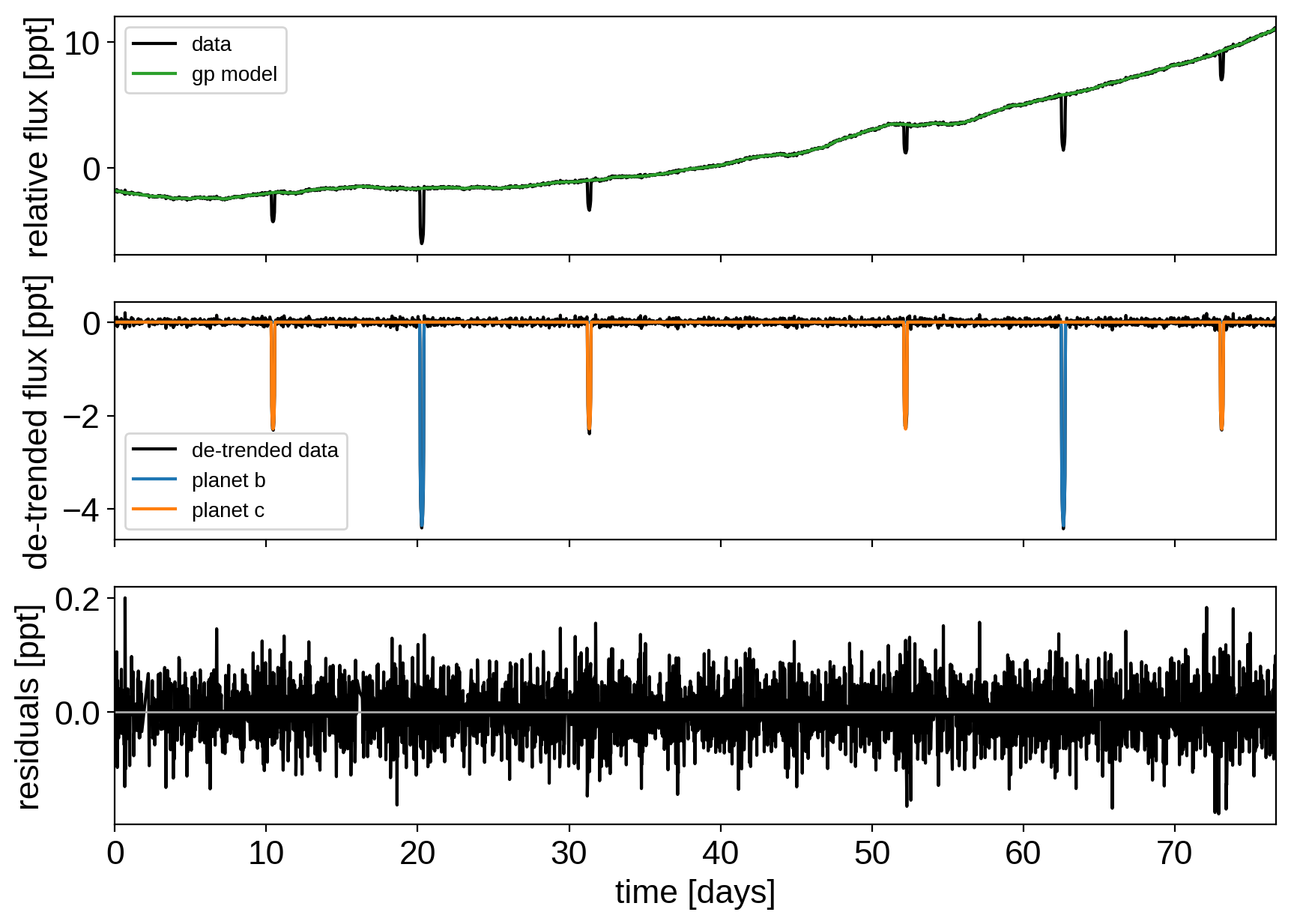Great! Now we’re ready to sample.

## Sampling¶

The sampling for this model is the same as for all the previous tutorials, but it takes a bit longer (about 2 hours on my laptop). This is partly because the model is more expensive to compute than the previous ones and partly because there are some non-affine degeneracies in the problem (for example between impact parameter and eccentricity). It might be worth thinking about reparameterizations (in terms of duration instead of eccentricity), but that’s beyond the scope of this tutorial. Besides, using more traditional MCMC methods, this would have taken a lot more than 2 hours to get >1000 effective samples!

np.random.seed(123)
with model:
trace = pm.sample(
tune=6000,
draws=3000,
start=map_soln,
chains=4,
step=xo.get_dense_nuts_step(target_accept=0.9),
)

Multiprocess sampling (4 chains in 4 jobs)
NUTS: [logSw4, logw0, logs2, trend, logs_rv, omega, ecc_frac, ecc_sigma_rayleigh, ecc_sigma_gauss, ecc, b, logr, t0, logP, logm, r_star, m_star, u_star, mean]
Sampling 4 chains: 100%|██████████| 36000/36000 [1:12:12<00:00,  1.84draws/s]
There were 81 divergences after tuning. Increase target_accept or reparameterize.
There were 50 divergences after tuning. Increase target_accept or reparameterize.
There were 46 divergences after tuning. Increase target_accept or reparameterize.
There were 58 divergences after tuning. Increase target_accept or reparameterize.
The number of effective samples is smaller than 10% for some parameters.


Let’s look at the convergence diagnostics for some of the key parameters:

pm.summary(trace, varnames=["period", "r_pl", "m_pl", "ecc", "omega", "b"])

mean sd mc_error hpd_2.5 hpd_97.5 n_eff Rhat
period__0 42.363456 0.000396 0.000004 42.362689 42.364234 10642.409433 0.999895
period__1 20.885338 0.000202 0.000002 20.884931 20.885721 8645.015917 1.000072
r_pl__0 0.077521 0.004554 0.000111 0.068177 0.085870 1941.768212 1.000340
r_pl__1 0.055888 0.003533 0.000094 0.048931 0.062613 1647.351091 1.000157
m_pl__0 27.223719 6.014221 0.065348 15.693746 39.294948 6928.785328 1.000213
m_pl__1 22.130263 4.413712 0.047499 13.497093 31.012766 6670.330514 1.000238
ecc__0 0.039703 0.034472 0.000653 0.000002 0.107371 2786.435033 1.000697
ecc__1 0.107645 0.093834 0.002300 0.000002 0.293460 1503.452582 1.000982
omega__0 0.143650 2.014668 0.035205 -3.134686 2.927897 2847.120109 1.000033
omega__1 -0.347757 1.034447 0.021766 -2.506963 2.330928 2172.343269 1.000205
b__0 0.588049 0.050939 0.001340 0.485199 0.669322 1440.172010 1.001588
b__1 0.538854 0.111060 0.003621 0.311607 0.726459 1048.071567 1.000011

As you see, the effective number of samples for the impact parameters and eccentricites are lower than for the other parameters. This is because of the correlations that I mentioned above:

import corner

varnames = ["b", "ecc"]
samples = pm.trace_to_dataframe(trace, varnames=varnames)
fig = corner.corner(samples);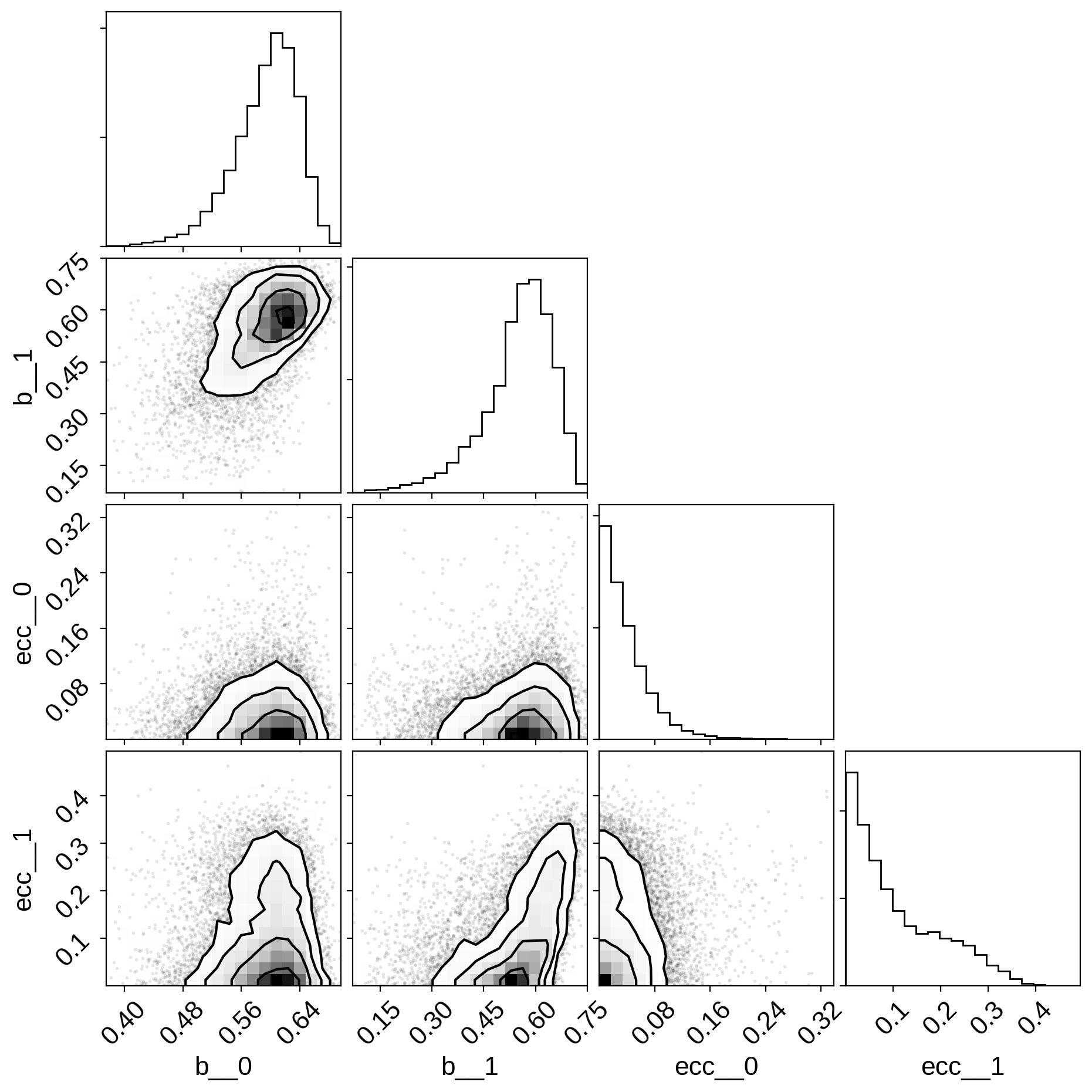## Phase plots¶

Finally, as in the Radial velocity fitting and Transit fitting tutorials, we can make folded plots of the transits and the radial velocities and compare to the posterior model predictions. (Note: planets b and c in this tutorial are swapped compared to the labels from Petigura et al. (2016))

for n, letter in enumerate("bc"):
plt.figure()

# Compute the GP prediction
gp_mod = np.median(trace["gp_pred"] + trace["mean"][:, None], axis=0)

# Get the posterior median orbital parameters
p = np.median(trace["period"][:, n])
t0 = np.median(trace["t0"][:, n])

# Compute the median of posterior estimate of the contribution from
# the other planet. Then we can remove this from the data to plot
# just the planet we care about.
other = np.median(trace["light_curves"][:, :, (n + 1) % 2], axis=0)

# Plot the folded data
x_fold = (x[mask] - t0 + 0.5 * p) % p - 0.5 * p
plt.plot(x_fold, y[mask] - gp_mod - other, ".k", label="data", zorder=-1000)

# Plot the folded model
inds = np.argsort(x_fold)
inds = inds[np.abs(x_fold)[inds] < 0.3]
pred = trace["light_curves"][:, inds, n]
pred = np.percentile(pred, [16, 50, 84], axis=0)
plt.plot(x_fold[inds], pred, color="C1", label="model")
art = plt.fill_between(
x_fold[inds], pred, pred, color="C1", alpha=0.5, zorder=1000
)
art.set_edgecolor("none")

# Annotate the plot with the planet's period
txt = "period = {0:.4f} +/- {1:.4f} d".format(
np.mean(trace["period"][:, n]), np.std(trace["period"][:, n])
)
plt.annotate(
txt,
(0, 0),
xycoords="axes fraction",
xytext=(5, 5),
textcoords="offset points",
ha="left",
va="bottom",
fontsize=12,
)

plt.legend(fontsize=10, loc=4)
plt.xlim(-0.5 * p, 0.5 * p)
plt.xlabel("time since transit [days]")
plt.ylabel("de-trended flux")
plt.title("K2-24{0}".format(letter))
plt.xlim(-0.3, 0.3)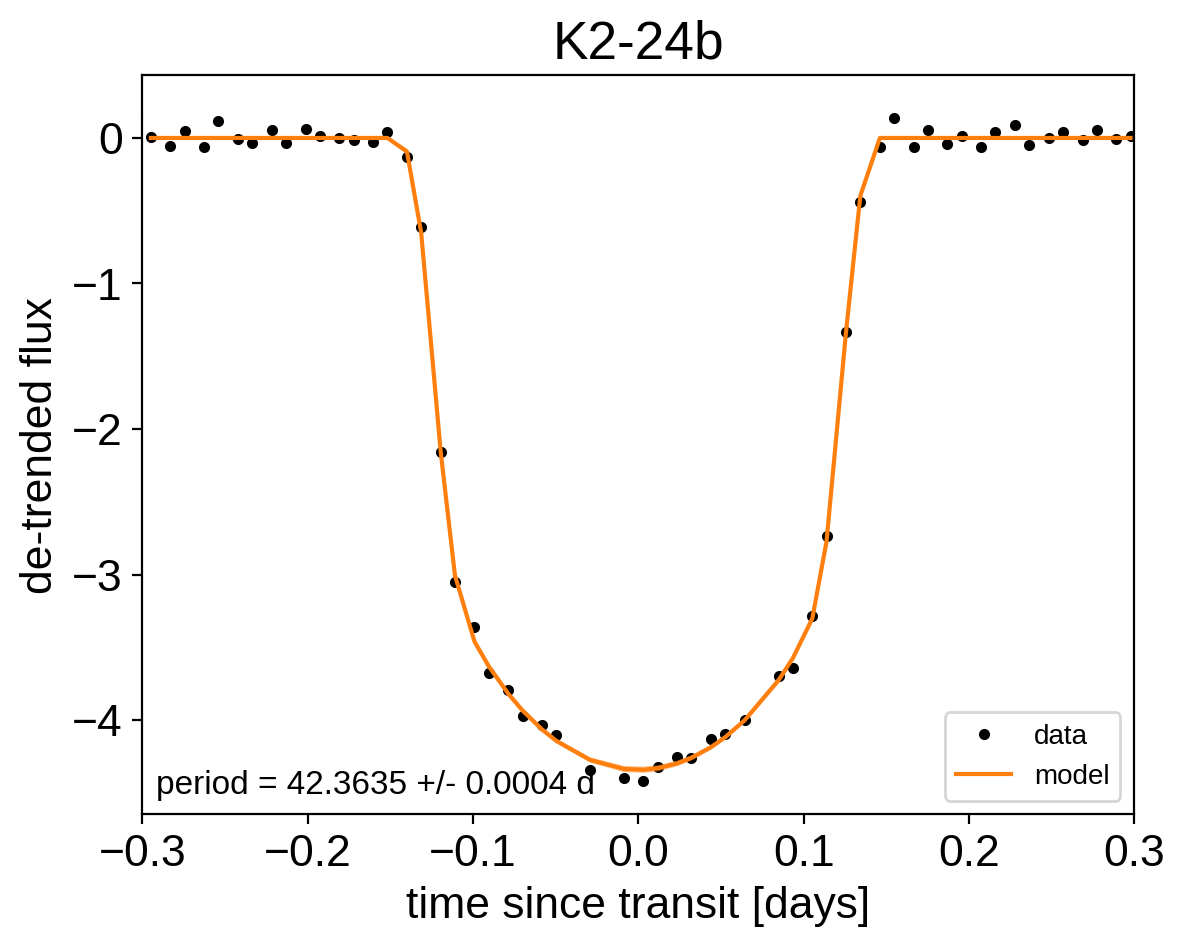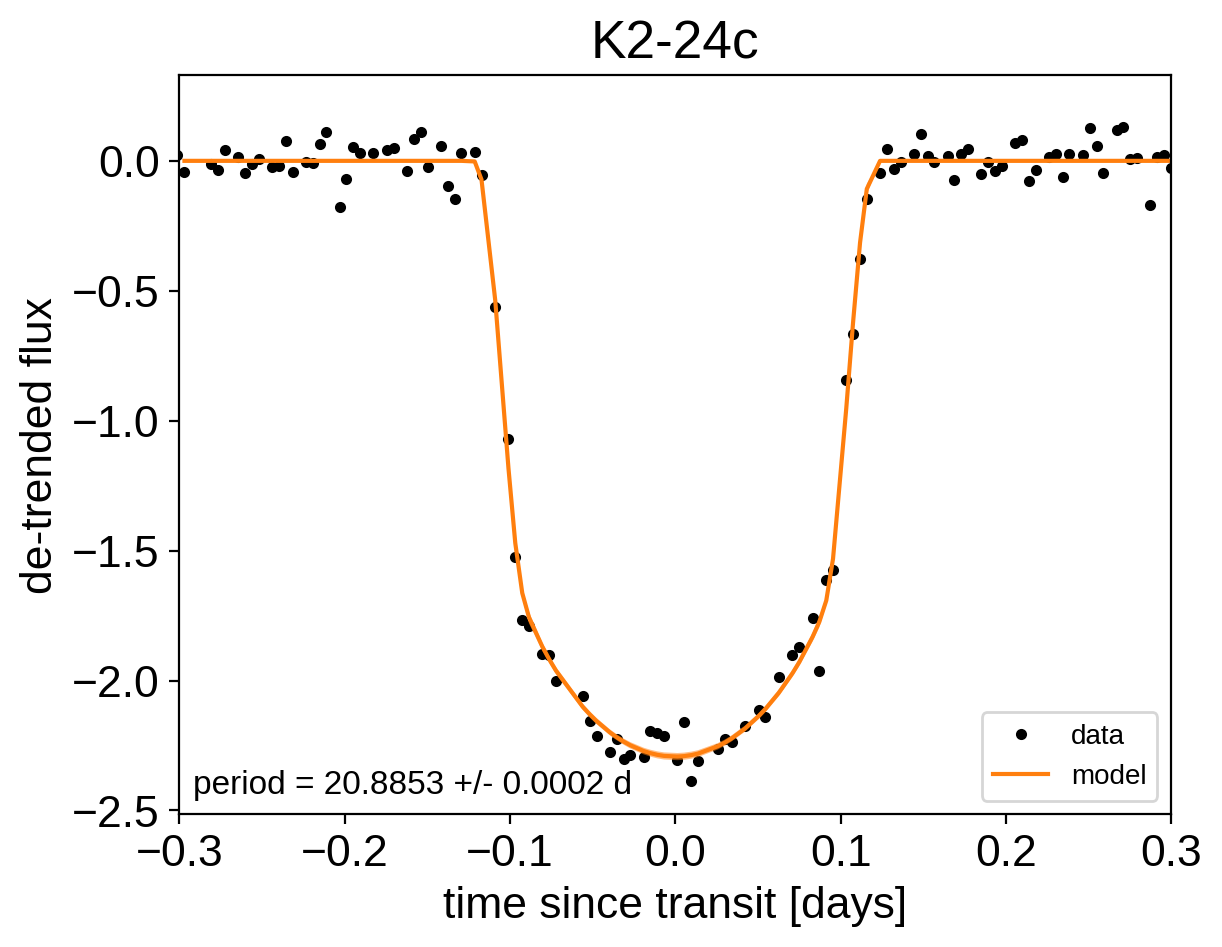for n, letter in enumerate("bc"):
plt.figure()

# Get the posterior median orbital parameters
p = np.median(trace["period"][:, n])
t0 = np.median(trace["t0"][:, n])

# Compute the median of posterior estimate of the background RV
# and the contribution from the other planet. Then we can remove
# this from the data to plot just the planet we care about.
other = np.median(trace["vrad"][:, :, (n + 1) % 2], axis=0)
other += np.median(trace["bkg"], axis=0)

# Plot the folded data
x_fold = (x_rv - t0 + 0.5 * p) % p - 0.5 * p
plt.errorbar(x_fold, y_rv - other, yerr=yerr_rv, fmt=".k", label="data")

# Compute the posterior prediction for the folded RV model for this
# planet
t_fold = (t_rv - t0 + 0.5 * p) % p - 0.5 * p
inds = np.argsort(t_fold)
pred = np.percentile(trace["vrad_pred"][:, inds, n], [16, 50, 84], axis=0)
plt.plot(t_fold[inds], pred, color="C1", label="model")
art = plt.fill_between(t_fold[inds], pred, pred, color="C1", alpha=0.3)
art.set_edgecolor("none")

plt.legend(fontsize=10)
plt.xlim(-0.5 * p, 0.5 * p)
plt.xlabel("phase [days]")
plt.title("K2-24{0}".format(letter));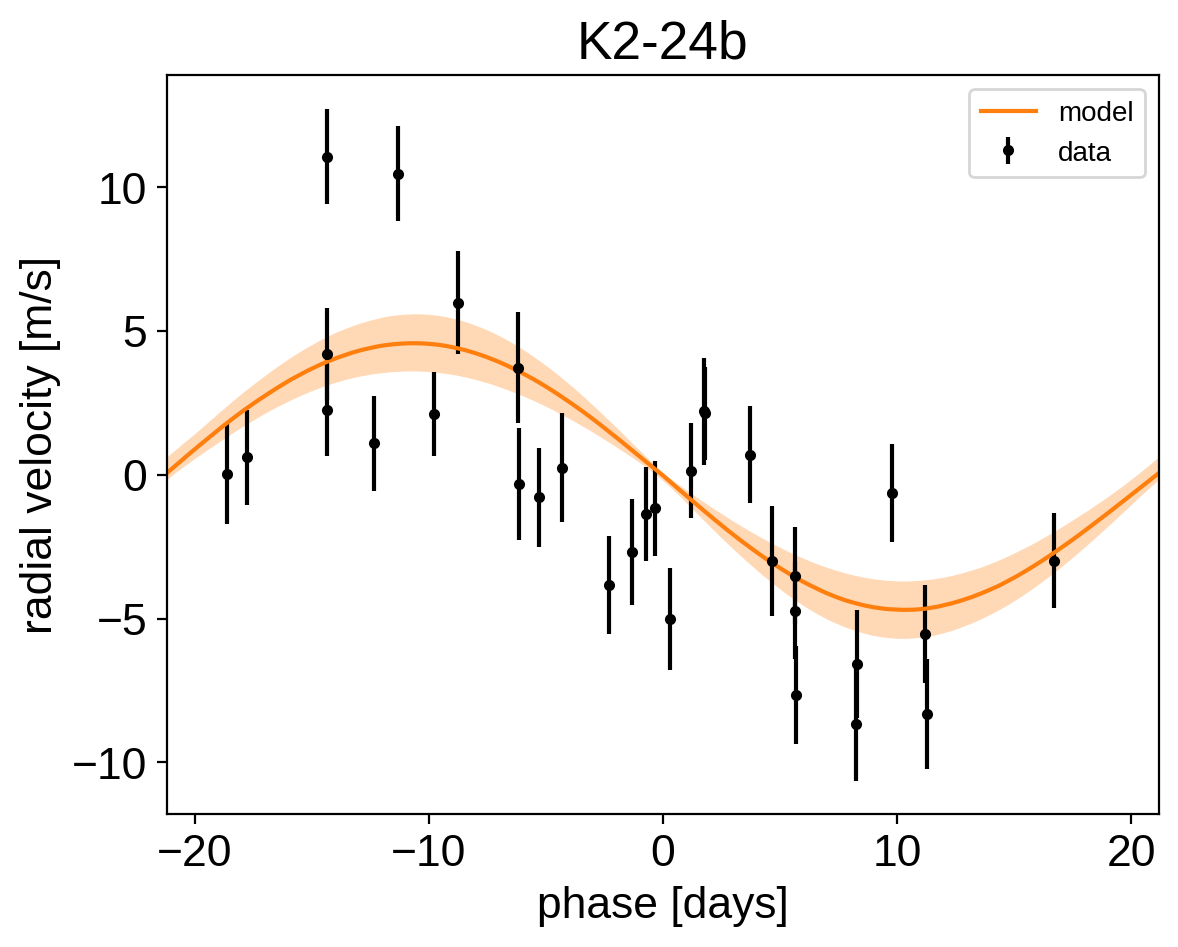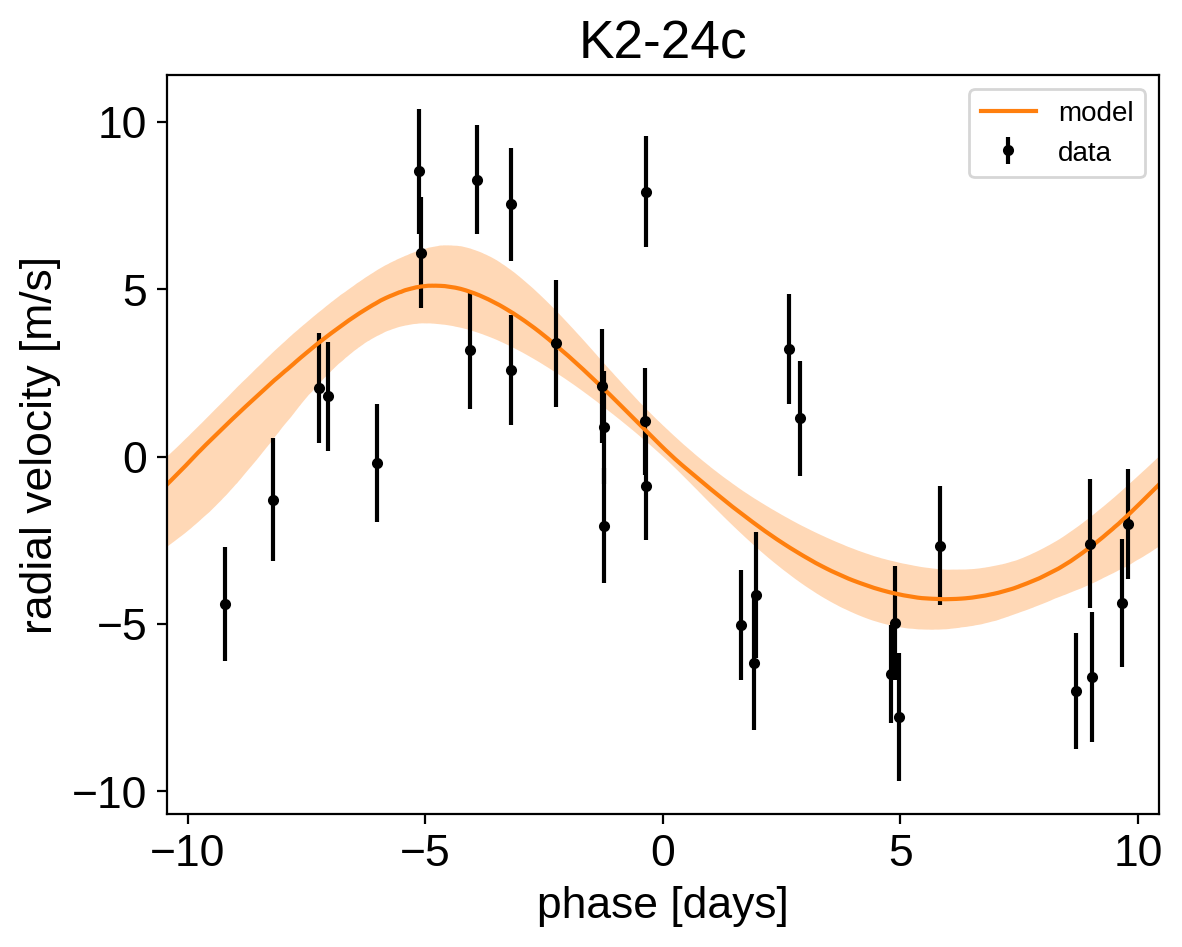We can also compute the posterior constraints on the planet densities.

volume = 4 / 3 * np.pi * trace["r_pl"] ** 3
density = u.Quantity(trace["m_pl"] / volume, unit=u.M_earth / u.R_sun ** 3)
density = density.to(u.g / u.cm ** 3).value

bins = np.linspace(0, 1.1, 45)
for n, letter in enumerate("bc"):
plt.hist(
density[:, n],
bins,
histtype="step",
lw=2,
label="K2-24{0}".format(letter),
density=True,
)
plt.yticks([])
plt.legend(fontsize=12)
plt.xlim(bins, bins[-1])
plt.xlabel("density [g/cc]")
plt.ylabel("posterior density");